# Algebra 1 : How to factor an equation

## Example Questions

2 Next →

### Example Question #2371 : Algebra 1

Find the solutions to the following equation.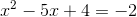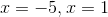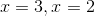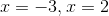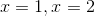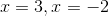Explanation:

The first step in solving the equation is to addto both sides so that we can set the new equation equal toso that we can factor it.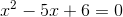We now need to factor it. We need to find two numbers,and, such that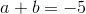and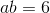.

Those two numbers are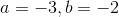Our new factored expression becomes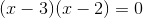Now we can easily identify that the values that satisfy this equation are.

### Example Question #11 : How To Factor An Equation

Solve by completing the square: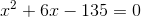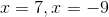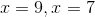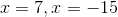no real solution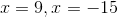Explanation:

In order to set this up for completing the square, we need to move the 135 to the other side: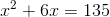Now the equation is in the form: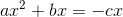To complete the square we need to add to both sides the folowing value: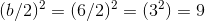So we need to add 9 to both sides of the equation: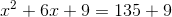Now we can factor the left side and simplify the left side: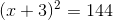Now we need to take the square root of both sides: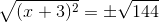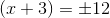**NOTE: Don't foget to add the plus or minus symbol. We add this becase there are two values we can square to get 144: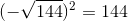and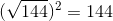**End note

Now we can split into two equations and solve for x: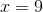and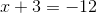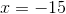So our solution is:### Example Question #12 : How To Factor An Equation

Factor: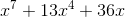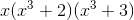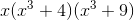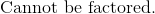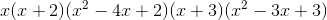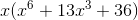Explanation:

There is a common variablein each term.  Pull this out as a common factor.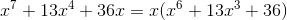Factor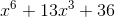.

The common factors that will achieve the middle term and will have a product of 36 is four and nine.  Write the binomials.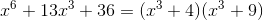The cubics here cannot be simplified any further.

The answer is:2 Next →